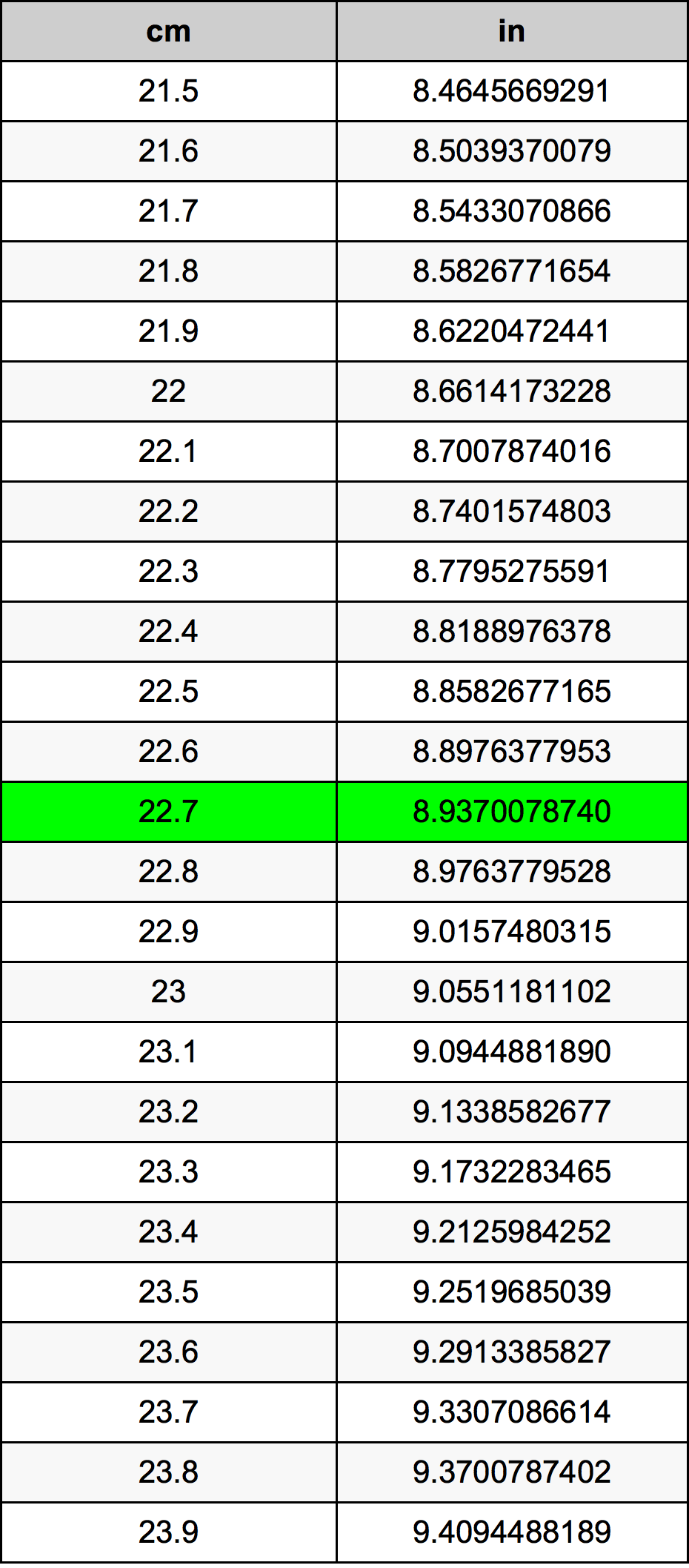Cm To Inches

# 22.7 cm to in22.7 Centimeters to Inches

cm
=
in

## How to convert 22.7 centimeters to inches?

 22.7 cm * 0.3937007874 in = 8.937007874 in 1 cm
A common question is How many centimeter in 22.7 inch? And the answer is 57.658 cm in 22.7 in. Likewise the question how many inch in 22.7 centimeter has the answer of 8.937007874 in in 22.7 cm.

## How much are 22.7 centimeters in inches?

22.7 centimeters equal 8.937007874 inches (22.7cm = 8.937007874in). Converting 22.7 cm to in is easy. Simply use our calculator above, or apply the formula to change the length 22.7 cm to in.

## Convert 22.7 cm to common lengths

UnitUnit of length
Nanometer227000000.0 nm
Micrometer227000.0 µm
Millimeter227.0 mm
Centimeter22.7 cm
Inch8.937007874 in
Foot0.7447506562 ft
Yard0.2482502187 yd
Meter0.227 m
Kilometer0.000227 km
Mile0.0001410513 mi
Nautical mile0.0001225702 nmi

## What is 22.7 centimeters in in?

To convert 22.7 cm to in multiply the length in centimeters by 0.3937007874. The 22.7 cm in in formula is [in] = 22.7 * 0.3937007874. Thus, for 22.7 centimeters in inch we get 8.937007874 in.

## 22.7 Centimeter Conversion Table## Alternative spelling

22.7 Centimeters to Inch, 22.7 Centimeters in Inch, 22.7 Centimeter to Inches, 22.7 Centimeter in Inches, 22.7 cm to Inch, 22.7 cm in Inch, 22.7 cm to in, 22.7 cm in in, 22.7 Centimeters to in, 22.7 Centimeters in in, 22.7 Centimeters to Inches, 22.7 Centimeters in Inches, 22.7 Centimeter to Inch, 22.7 Centimeter in Inch